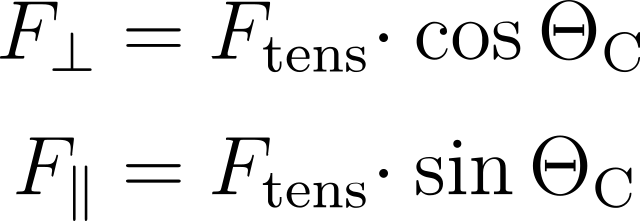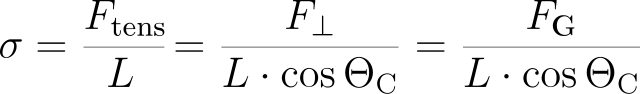Wilhelmy plate method# Wilhelmy plate method

Surface and interfacial tensions can be measured with a dynamic contact angle measuring instrument and tensiometer of the DCAT series. For this purpose the weight of a liquid lamella that forms when a measuring body is wetted is being measured. Accordingly, the most important part of a tensiometer is a highly precise balance to which the measuring bodies are being attached. A vessel with the liquid of interest is placed under the balance on a height-adjustable table (see figure 1).

The most common method to measure the surface/interfacial tension is the Wilhelmy plate method. A Wilhelmy plate is a thin, generally rectangular plate made of iridium–platinum with a few centimetres in length and height. The material is chosen for reasons of good wetting when contacting a liquid. In this case a lamella snaps on whose shape is determined by the interfacial tension of the liquid. The volume and hence the weight of the formed lamella furthermore depends on the wetted length L = 2 · b + 2 · l of the Wilhelmy plate (see figure 2).

At the three-phase contact line a tension force Ftens acts tangentially to the liquid surface. It can be split in a force parallel and a force perpendicular to the undisturbed liquid surface:Figure 1: Schematic setup of a tensiometer

The balance, which is located in z-direction, only feels the perpendicular part of the tension force which just matches the gravitational force FG of the formed lamella. (Keep in mind that the gravitational force of the measuring body is irrelevant because the balance of the tensiometer is tared with attached measuring body at the beginning of a measurement.) With the definition of the surface tension the Wilhelmy equation is obtained as:Assuming that the Wilhelmy plate is completely wetted (contact angle 0°) the equation simplifies (cos(0°)=1), which enables a direct determination of the surface tension knowing the plate dimensions and the measured gravitational force.

In practice, one ensures a complete wetting not only by using iridium–platinum as plate material but also by a specific measurement procedure: The Wilhelmy plate is first dipped into the liquid and then pulled back to the position of first contact (meaning the table with the sample vessel is moved accordingly).

The interfacial tension between two liquids can be determined with the Wilhelmy plate method, analogously to the surface tension. For this purpose the gravitational force of the lamella that forms when the Wilhelmy plate stands at the interface between the two liquids has to be measured. The plate itself is then located inside the upper (less dense/lighter) phase where it experiences a buoyancy force Fbuoy = ρgV with the local gravity acceleration g, liquid density ρ and displaced volume V.

A convenient way to compensate for this effect is to initially submerge the Wilhelmy plate completely in the lighter phase and to tare the balance in this state. Afterwards the vessel with the lighter phase is removed and a vessel with the heavier phase is placed on the sample table. To form the interfacial lamella the Wilhelmy plate is then completely submerged into the heavier phase, on top of which one subsequently carefully fills a layer of the lighter phase. Finally, the Wilhelmy plate is pulled back to the interface. The weight that is measured now is equal to the gravitational force of the interfacial lamella and the interfacial tension can be calculated according to the Wilhelmy equation.

Figure 2: Wilhelmy plate with liquid lamella

# Chapters

Using the following buttons you can navigate between the individual chapters of our knowledge-base Understanding Interfaces